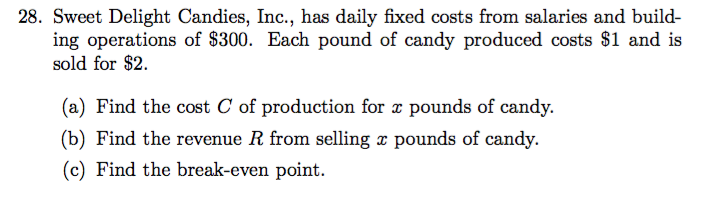# 28. Sweet Delight Candies, Inc., has daily fixed costs from salaries and build-ing operations of \$300. Each pound of candy produced costs \$1 and issold for \$2(a) Find the cost C of production for x pounds of candy.(b) Find the revenue R from selling x pounds of candy.(c) Find the break-even point

Questionhelp_outlineImage Transcriptionclose28. Sweet Delight Candies, Inc., has daily fixed costs from salaries and build- ing operations of \$300. Each pound of candy produced costs \$1 and is sold for \$2 (a) Find the cost C of production for x pounds of candy. (b) Find the revenue R from selling x pounds of candy. (c) Find the break-even point fullscreen
check_circleExpert Solution
Step 1

(a) Let the number of pounds of candies be x.

The total production cost for x pounds of candy is = 300 + (1)(x), that is C = 300 + x.

Step 2

(b) Here, since each pound of candy is sold for \$2, the total revenue from selling x pounds of candy is R = 2x.

Step 3

(c) The break-even point is computed by equating the above two equations.

...

### Want to see the full answer?

See Solution

#### Want to see this answer and more?

Solutions are written by subject experts who are available 24/7. Questions are typically answered within 1 hour*

See Solution
*Response times may vary by subject and question
Tagged in

### Calculus